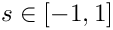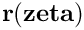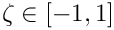oomph::QuarterPipeDomain Class Reference

Domain representing a quarter pipe. More...

#include <quarter_pipe_domain.h>Inheritance diagram for oomph::QuarterPipeDomain:

## Public Types

typedef double(* AxialSpacingFctPt) (const double &xi)
Typedef for function pointer for function that implements axial spacing of macro elements. More...

## Public Member Functions

QuarterPipeDomain (const unsigned &ntheta, const unsigned &nr, const unsigned &nz, const double &rmin, const double &rmax, const double &length)
Constructor: Pass number of elements in various directions, the inner and outer radius and the length of the tube. More...

QuarterPipeDomain (const QuarterPipeDomain &)=delete
Broken copy constructor. More...

void operator= (const QuarterPipeDomain &)=delete
Broken assignment operator. More...

~QuarterPipeDomain ()
Destructor: More...

AxialSpacingFctPtaxial_spacing_fct_pt ()
Function pointer for function that implements axial spacing of macro elements. More...

double axial_spacing_fct (const double &xi)
Function that implements axial spacing of macro elements. More...

void macro_element_boundary (const unsigned &t, const unsigned &i_macro, const unsigned &i_direct, const Vector< double > &s, Vector< double > &f)
Vector representation of the i_macro-th macro element boundary i_direct (U/D/L/R/F/B) at time level t (t=0: present; t>0: previous): f(s). More...Public Member Functions inherited from oomph::Domain
Domain ()
Constructor. More...

Domain (const Domain &)=delete
Broken copy constructor. More...

void operator= (const Domain &)=delete
Broken assignment operator. More...

virtual ~Domain ()
Destructor: Strictly speaking, whoever creates an object dynamically should be responsible for the cleanup of said object but it makes sense here for the Domain to generically kill any MacroElements left over in the MacroElement container (if it hasn't already been done in the derived class) to avoid memory leaks. More...

MacroElementmacro_element_pt (const unsigned &i)

unsigned nmacro_element ()
Number of macro elements in domain. More...

void output (const std::string &filename, const unsigned &nplot)
Output macro elements. More...

void output (std::ostream &outfile, const unsigned &nplot)
Output macro elements. More...

virtual void macro_element_boundary (const double &t, const unsigned &i_macro, const unsigned &i_direct, const Vector< double > &s, Vector< double > &f)
Vector representation of the i_macro-th macro element boundary i_direct (e.g. N/S/W/E in 2D) at continuous time, t. More...

void macro_element_boundary (const unsigned &i_macro, const unsigned &i_direct, const Vector< double > &s, Vector< double > &f)
Vector representation of the i_macro-th macro element boundary i_direct (e.g. N/S/W/E in 2D) at current time: f(s). More...

void output_macro_element_boundaries (const std::string &filename, const unsigned &nplot)
Output all macro element boundaries as tecplot zones. More...

void output_macro_element_boundaries (std::ostream &outfile, const unsigned &nplot)
Output all macro element boundaries as tecplot zones. More...

virtual void dmacro_element_boundary (const unsigned &t, const unsigned &i_macro, const unsigned &i_direct, const Vector< double > &s, Vector< double > &f)
Vector representation of the i_macro-th macro element boundary derivatives i_direct (e.g. N/S/W/E in 2D) at time level t (t=0: present; t>0: previous): f(s). Broken virtual. More...

virtual void dmacro_element_boundary (const double &t, const unsigned &i_macro, const unsigned &i_direct, const Vector< double > &s, Vector< double > &f)
Vector representation of the i_macro-th macro element boundary derivatives i_direct (e.g. N/S/W/E in 2D) at continuous time level t. Broken virtual. More...

void dmacro_element_boundary (const unsigned &i_macro, const unsigned &i_direct, const Vector< double > &s, Vector< double > &f)
Vector representation of the i_macro-th macro element boundary derivatives i_direct (e.g. N/S/W/E in 2D) at current time: f(s). More...

virtual void d2macro_element_boundary (const unsigned &t, const unsigned &i_macro, const unsigned &i_direct, const Vector< double > &s, Vector< double > &f)
Vector representation of the i_macro-th macro element boundary second derivatives i_direct (e.g. N/S/W/E in 2D) at time level t (t=0: present; t>0: previous): f(s). Broken virtual. More...

virtual void d2macro_element_boundary (const double &t, const unsigned &i_macro, const unsigned &i_direct, const Vector< double > &s, Vector< double > &f)
Vector representation of the i_macro-th macro element boundary seocond derivatives i_direct (e.g. N/S/W/E in 2D) at continuous time level t. Broken virtual. More...

void d2macro_element_boundary (const unsigned &i_macro, const unsigned &i_direct, const Vector< double > &s, Vector< double > &f)
Vector representation of the i_macro-th macro element boundary second derivatives i_direct (e.g. N/S/W/E in 2D) at current time: f(s). More...

## Private Member Functions

void r_U (const unsigned &t, const Vector< double > &zeta, Vector< double > &f, const double &rmin, const double &rmax, const double &thetamin, const double &thetamax, const double &zmin, const double &zmax)
Boundary of macro element zeta. More...

void r_L (const unsigned &t, const Vector< double > &zeta, Vector< double > &f, const double &rmin, const double &rmax, const double &thetamin, const double &thetamax, const double &zmin, const double &zmax)
Boundary of macro element zeta. More...

void r_D (const unsigned &t, const Vector< double > &zeta, Vector< double > &f, const double &rmin, const double &rmax, const double &thetamin, const double &thetamax, const double &zmin, const double &zmax)
Boundary of macro element zeta. More...

void r_R (const unsigned &t, const Vector< double > &zeta, Vector< double > &f, const double &rmin, const double &rmax, const double &thetamin, const double &thetamax, const double &zmin, const double &zmax)
Boundary of macro element zeta. More...

void r_F (const unsigned &t, const Vector< double > &zeta, Vector< double > &f, const double &rmin, const double &rmax, const double &thetamin, const double &thetamax, const double &zmin, const double &zmax)
Boundary of macro element zeta. More...

void r_B (const unsigned &t, const Vector< double > &zeta, Vector< double > &f, const double &rmin, const double &rmax, const double &thetamin, const double &thetamax, const double &zmin, const double &zmax)
Boundary of macro element zeta. More...

## Static Private Member Functions

static double default_axial_spacing_fct (const double &xi)
Default for function that implements axial spacing of macro elements. More...

## Private Attributes

unsigned Ntheta
Number of elements azimuthal direction. More...

unsigned Nr
Number of elements radial direction. More...

unsigned Nz
Number of elements axial direction. More...

double Rmin

double Rmax

double Length
Length. More...

GeomObjectOuter_boundary_cross_section_pt
Geom object representing the outer boundary of the cross section. More...

GeomObjectInner_boundary_cross_section_pt
Geom object representing the inner boundary of the cross section. More...

AxialSpacingFctPt Axial_spacing_fct_pt
Function pointer for function that implements axial spacing of macro elements. More...Protected Attributes inherited from oomph::Domain
Vector< MacroElement * > Macro_element_pt
Vector of pointers to macro elements. More...

## Detailed Description

Domain representing a quarter pipe.

Definition at line 40 of file quarter_pipe_domain.h.

## ◆ AxialSpacingFctPt

 typedef double(* oomph::QuarterPipeDomain::AxialSpacingFctPt) (const double &xi)

Typedef for function pointer for function that implements axial spacing of macro elements.

Definition at line 93 of file quarter_pipe_domain.h.

## ◆ QuarterPipeDomain() [1/2]

 oomph::QuarterPipeDomain::QuarterPipeDomain ( const unsigned & ntheta, const unsigned & nr, const unsigned & nz, const double & rmin, const double & rmax, const double & length )
inline

Constructor: Pass number of elements in various directions, the inner and outer radius and the length of the tube.

Definition at line 45 of file quarter_pipe_domain.h.

## ◆ QuarterPipeDomain() [2/2]

 oomph::QuarterPipeDomain::QuarterPipeDomain ( const QuarterPipeDomain & )
delete

Broken copy constructor.

## ◆ ~QuarterPipeDomain()

 oomph::QuarterPipeDomain::~QuarterPipeDomain ( )
inline

Destructor:

Definition at line 84 of file quarter_pipe_domain.h.

References Inner_boundary_cross_section_pt, and Outer_boundary_cross_section_pt.

## ◆ axial_spacing_fct()

 double oomph::QuarterPipeDomain::axial_spacing_fct ( const double & xi )
inline

Function that implements axial spacing of macro elements.

Definition at line 104 of file quarter_pipe_domain.h.

References Axial_spacing_fct_pt.

Referenced by macro_element_boundary().

## ◆ axial_spacing_fct_pt()

 AxialSpacingFctPt & oomph::QuarterPipeDomain::axial_spacing_fct_pt ( )
inline

Function pointer for function that implements axial spacing of macro elements.

Definition at line 97 of file quarter_pipe_domain.h.

References Axial_spacing_fct_pt.

## ◆ default_axial_spacing_fct()

 static double oomph::QuarterPipeDomain::default_axial_spacing_fct ( const double & xi )
inlinestaticprivate

Default for function that implements axial spacing of macro elements.

Definition at line 151 of file quarter_pipe_domain.h.

## ◆ macro_element_boundary()

 void oomph::QuarterPipeDomain::macro_element_boundary ( const unsigned & t, const unsigned & i_macro, const unsigned & i_direct, const Vector< double > & s, Vector< double > & f )
virtual

Vector representation of the i_macro-th macro element boundary i_direct (U/D/L/R/F/B) at time level t (t=0: present; t>0: previous): f(s).

Vector representation of the imacro-th macro element boundary idirect (U/D/L/R/F/B) at time level t: f(s)

Implements oomph::Domain.

Definition at line 230 of file quarter_pipe_domain.h.

## ◆ operator=()

 void oomph::QuarterPipeDomain::operator= ( const QuarterPipeDomain & )
delete

Broken assignment operator.

## ◆ r_B()

 void oomph::QuarterPipeDomain::r_B ( const unsigned & t, const Vector< double > & zeta, Vector< double > & f, const double & rmin, const double & rmax, const double & thetamin, const double & thetamax, const double & zmin, const double & zmax )
private

Boundary of macro element zeta.

Back face of a macro element.

Definition at line 490 of file quarter_pipe_domain.h.

Referenced by macro_element_boundary().

## ◆ r_D()

 void oomph::QuarterPipeDomain::r_D ( const unsigned & t, const Vector< double > & zeta, Vector< double > & f, const double & rmin, const double & rmax, const double & thetamin, const double & thetamax, const double & zmin, const double & zmax )
private

Boundary of macro element zeta.

Left face of a macro element.

Definition at line 387 of file quarter_pipe_domain.h.

Referenced by macro_element_boundary().

## ◆ r_F()

 void oomph::QuarterPipeDomain::r_F ( const unsigned & t, const Vector< double > & zeta, Vector< double > & f, const double & rmin, const double & rmax, const double & thetamin, const double & thetamax, const double & zmin, const double & zmax )
private

Boundary of macro element zeta.

Front face of a macro element.

Definition at line 455 of file quarter_pipe_domain.h.

Referenced by macro_element_boundary().

## ◆ r_L()

 void oomph::QuarterPipeDomain::r_L ( const unsigned & t, const Vector< double > & zeta, Vector< double > & f, const double & rmin, const double & rmax, const double & thetamin, const double & thetamax, const double & zmin, const double & zmax )
private

Boundary of macro element zeta.

Left face of a macro element.

Definition at line 304 of file quarter_pipe_domain.h.

Referenced by macro_element_boundary().

## ◆ r_R()

 void oomph::QuarterPipeDomain::r_R ( const unsigned & t, const Vector< double > & zeta, Vector< double > & f, const double & rmin, const double & rmax, const double & thetamin, const double & thetamax, const double & zmin, const double & zmax )
private

Boundary of macro element zeta.

Right face of a macro element.

Definition at line 345 of file quarter_pipe_domain.h.

Referenced by macro_element_boundary().

## ◆ r_U()

 void oomph::QuarterPipeDomain::r_U ( const unsigned & t, const Vector< double > & zeta, Vector< double > & f, const double & rmin, const double & rmax, const double & thetamin, const double & thetamax, const double & zmin, const double & zmax )
private

Boundary of macro element zeta.

Right face of a macro element.

Definition at line 421 of file quarter_pipe_domain.h.

Referenced by macro_element_boundary().

## ◆ Axial_spacing_fct_pt

 AxialSpacingFctPt oomph::QuarterPipeDomain::Axial_spacing_fct_pt
private

Function pointer for function that implements axial spacing of macro elements.

Definition at line 147 of file quarter_pipe_domain.h.

Referenced by axial_spacing_fct(), and axial_spacing_fct_pt().

## ◆ Inner_boundary_cross_section_pt

 GeomObject* oomph::QuarterPipeDomain::Inner_boundary_cross_section_pt
private

Geom object representing the inner boundary of the cross section.

Definition at line 143 of file quarter_pipe_domain.h.

Referenced by QuarterPipeDomain(), r_B(), r_D(), r_F(), r_L(), r_R(), r_U(), and ~QuarterPipeDomain().

## ◆ Length

 double oomph::QuarterPipeDomain::Length
private

Length.

Definition at line 135 of file quarter_pipe_domain.h.

Referenced by macro_element_boundary().

## ◆ Nr

 unsigned oomph::QuarterPipeDomain::Nr
private

Definition at line 123 of file quarter_pipe_domain.h.

Referenced by macro_element_boundary().

## ◆ Ntheta

 unsigned oomph::QuarterPipeDomain::Ntheta
private

Number of elements azimuthal direction.

Definition at line 120 of file quarter_pipe_domain.h.

Referenced by macro_element_boundary().

## ◆ Nz

 unsigned oomph::QuarterPipeDomain::Nz
private

Number of elements axial direction.

Definition at line 126 of file quarter_pipe_domain.h.

Referenced by macro_element_boundary().

## ◆ Outer_boundary_cross_section_pt

 GeomObject* oomph::QuarterPipeDomain::Outer_boundary_cross_section_pt
private

Geom object representing the outer boundary of the cross section.

Definition at line 139 of file quarter_pipe_domain.h.

Referenced by QuarterPipeDomain(), r_B(), r_D(), r_F(), r_L(), r_R(), r_U(), and ~QuarterPipeDomain().

## ◆ Rmax

 double oomph::QuarterPipeDomain::Rmax
private

Definition at line 132 of file quarter_pipe_domain.h.

Referenced by macro_element_boundary(), r_B(), r_D(), r_F(), r_L(), r_R(), and r_U().

## ◆ Rmin

 double oomph::QuarterPipeDomain::Rmin
private